# Descriptive statistics in R

## Instructions:

Use the left and right arrow keys to navigate the presentation forward and backward respectively. You can also use the arrows at the bottom right of the screen to navigate with a mouse.

FAIR USE ACT DISCLAIMER:
This site is for educational purposes only. This website may contain copyrighted material, the use of which has not been specifically authorized by the copyright holders. The material is made available on this website as a way to advance teaching, and copyright-protected materials are used to the extent necessary to make this class function in a distance learning environment. The Fair Use Copyright Disclaimer is under section 107 of the Copyright Act of 1976, allowance is made for “fair use” for purposes such as criticism, comment, news reporting, teaching, scholarship, education and research.

## Outline

• The following topics will be covered in this lecture:
• An introduction to descriptive statistics in R
• Graphical representations
• Statistics of location and dispersion

## An introduction to descriptive statistics in R

• Let us consider an rv $$X$$ following a distribution $$F_θ$$ , $$X \sim F_\theta$$.

• To obtain a sample of $$n$$ observations one first constructs $$n$$ copies of the rv $$X$$, i.e.

• sample rvs $$X_1 , \cdots , X_n \sim F_\theta$$ which follow the same distribution $$F_\theta$$ as the original variable $$X$$.
• By way of analogy, this is how a binomial trial by replicating individual Bernouli trials, where each random variable represents an outcome of an individual Bernouli trial.
• All $$X_1 , \cdots , X_n$$ are often assumed to be independent, where “iid” refers to independent and identically distributed as above.

• When the real-world transpires, and each rv assigns a number to the outcome, we draw an observation $$x_i$$ of the rv $$X_i$$;

• this results in a random sample $$x_1 , \cdots , x_n$$.
• Note that these lower-case values $$x_1 , \cdots , x_n$$ are not rvs, as the outcome has already transpired, but instead just observations of the outcome.

• particularly, these can be used to estimate the unknown parameters $$\theta$$ (for normal distribution these are $$\mu$$ and $$\sigma$$).

### An introduction to descriptive statistics in R

• Note, because the observations are of a random process, the outcomes and thus the observations will change if we replicate the trial.

• If we compute the sample mean of the random variables, i.e., \begin{align} \overline{X} = \frac{1}{n}\sum_{i=1}^n X_i \end{align}

this is also a random variable, as it is simply a combination of random variables.

• However, computing this on the observations, after the outcome has transpired

\begin{align} \overline{x} = \frac{1}{n}\sum_{i=1}^n x_i \end{align}

is just a measure of the center of the cloud of the observations.

### Frequency tables

• Suppose we have observations $$\{x_i \}_{i=1}^n$$ of a rv $$X$$.

• Let $$\{a_j\}_{i=1}^k$$, denote all different realizations of $$X$$ in the sample.
• We will define two types of frequencies as follows:

• The absolute frequency of $$a_j$$, denoted by $$n(a_j)$$, is the number of occurrences of $$a_j$$ in the sample $$\{x_i\}_{i=1}^n$$.

• The relative frequency of $$a_j$$, denoted by $$h(a_j)$$, is the ratio of the absolute frequencies of $$a_j$$ and the sample size $$n$$, $h(a_j) = n(a_j)/n.$

• Clearly

$\sum_{j=1}^k h(a_j )= \sum_{j=1}^k \frac{n(a_j)}{n} = \frac{n}{n} = 1.$

### Frequency tables

• The function table() returns all observed values of the data along with their absolute frequencies.

• These can be used further to compute the relative frequencies by dividing by n.

• Let us consider the dataset chickwts, a data frame with 71 observations of 2 variables:

• weight, a numeric variable for the weight of the chicken, and
• feed, a factor for the type of feed.
• By using

table(chickwts$feed)   casein horsebean linseed meatmeal soybean sunflower 12 10 12 11 14 12  we get one line, stating the possible chicken feed, i.e. each possible observed value, and the absolute frequency of each type in the line below. ### Frequency tables • To put this into relative frequency, we can write table(chickwts$feed) / length(chickwts$feed)   casein horsebean linseed meatmeal soybean sunflower 0.1690141 0.1408451 0.1690141 0.1549296 0.1971831 0.1690141  • There are several methods of visualising the frequencies graphically. • Depending on which type of data is at our disposal, the adequate approach needs to be chosen carefully: • Bar Plot, Bar Diagram or Pie Chart for qualitative or discrete variables, and • the histogram for continuous (or quasi-continuous) variables. • Since the variable feed in dataset chickwts has only 6 distinct categories of feed, the frequencies can be conveniently shown using a Bar Plot, a Bar Diagram or a Pie Chart. ### Graphical frequency • Here we make a bar plot for the frequency table. par(cex.axis = 1.75, mai=c(1.5,1.5,.5,.5), mgp=c(3,0,0)) plot(table(chickwts$feed), ylab = expression(h(a[j])), xlab = "", cex.lab = 2)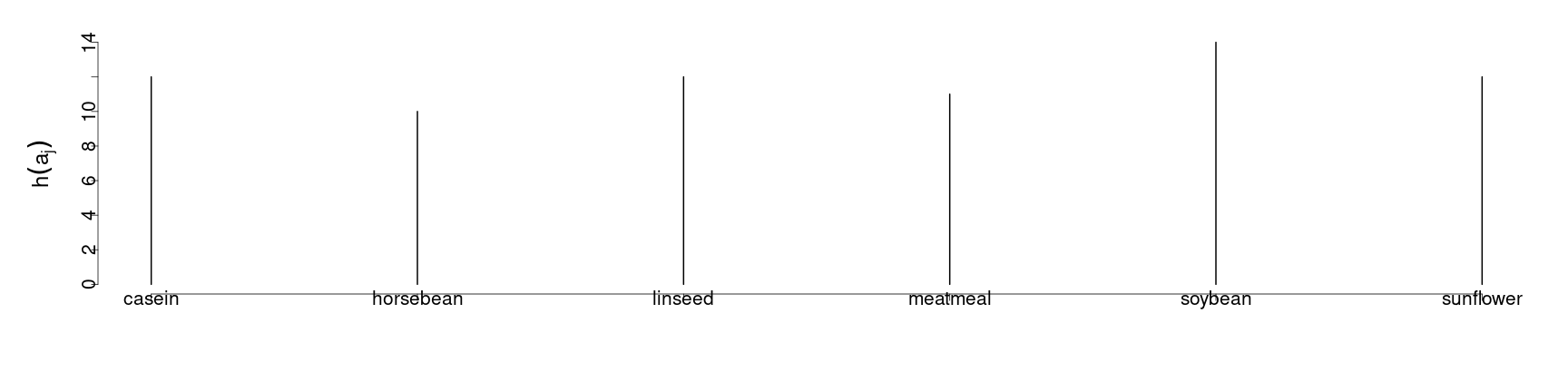### Graphical frequency

• Here we make a bar plot for the relative frequency table
par(cex.axis = 1.75, mai=c(1.5,1.5,.5,.5), mgp=c(3,0,0))
barplot(table(chickwts$feed)/length(chickwts$feed), space = 2, ylab = expression(h(a[j])), xlab = "", cex.lab = 2)
abline(h = 0)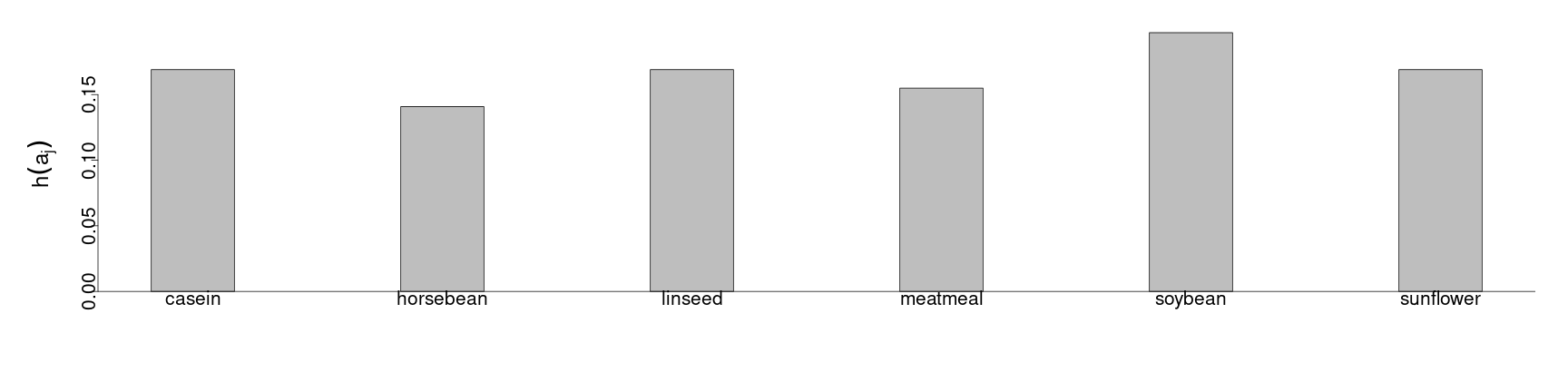### Graphical frequency

• Here we make a pie chart for the relative frequency table
par(cex = 1.75, mai=c(1.5,1.5,.5,.5), mgp=c(3,0,0))
pie(table(chickwts$feed)/length(chickwts$feed))### Empirical (Cumulative) Distribution Function

• The empirical cumulative distribution function (ecdf) is denoted by $$\hat{F}(x)$$ and describes the relative number of observations which are less than or equal to $$x$$ in the sample.

• We write $$\hat{F}(x)$$ with a hat because it is an estimator of the true cdf $F(x) = P(X \leq x).$

• $$\hat{F}(x)$$ converges almost surely to $$F(x)$$ when $$n$$, the sample size, goes to infinity due to the Strong Law of Large Numbers.

• The ecdf is a non-decreasing step function,

• i.e. it is a function which is constant except for jumps at a discrete set of points.
• The points where the jumps occur are the realizations of the rv and thus illustrate a general property of the cumulative distribution function:

• continuous at right and existing limit at left, which holds for all $$\hat{F}$$.
• This step function is given by ecdf(), which returns a function of class ecdf, which can be plotted using plot().

• Take for example the dataset Formaldehyde containing 6 observations on two variables:

1. carbohydrate (car) and
2. optical density (optden).

### Empirical (Cumulative) Distribution Function

• The ecdf for the subset Formaldehyde$car can be calculated as follows, d = ecdf(Formaldehyde$car)
summary(d)

Empirical CDF:    6 unique values with summary
Min. 1st Qu.  Median    Mean 3rd Qu.    Max.
0.1000  0.3500  0.5500  0.5167  0.6750  0.9000

• This allows us to see the empirical values for the CDF, as given by the ordered values in the sample.

### Empirical (Cumulative) Distribution Function

• We can plot this as
par(cex = 1.75, mai=c(1.5,1.5,.5,.5), mgp=c(3,0,0))
plot(d, ylab = expression(hat(F)(x)), xlab = "x", main = "")
abline(v = 0.5, lty = 2, lwd = 2)
text(0.71, 0.51, expression(hat(F)(0.5)), cex = 1.2)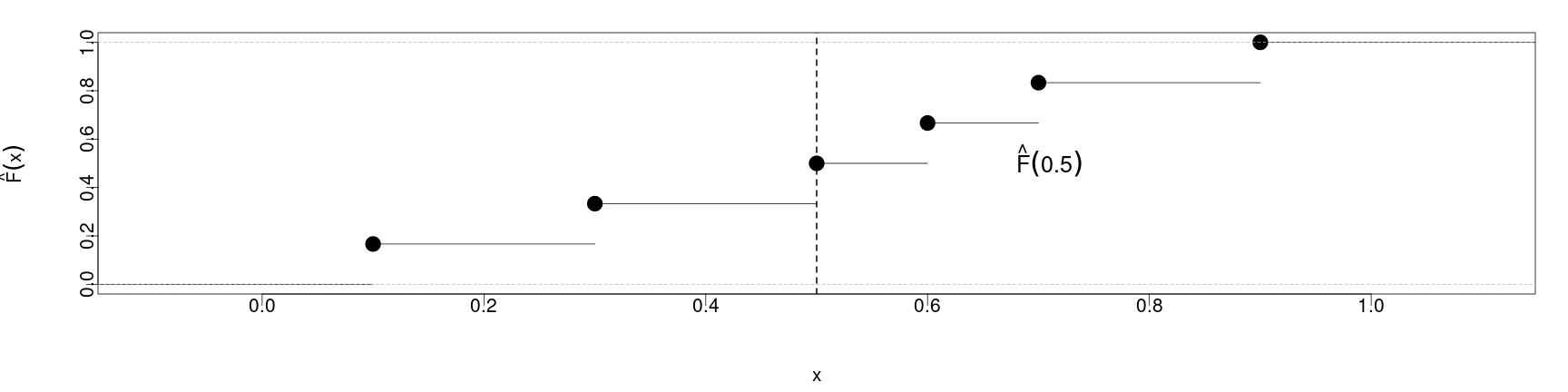• In the vertical axis, we have the relative frequency value defined by $$\hat{F}(x)$$ on the sample, i.e., \begin{align} \hat{F}(x) \approx \frac{\text{number of values less than }x\text{ in the sample}}{\text{total sample size }n} \end{align}

• Correspondingly, the horizontal axis represents the value $$x$$ in the functional argument.

### Empirical (Cumulative) Distribution Function

• We then can see the behavior of the step function where we have the six different measurements.
par(cex = 1.75, mai=c(1.5,1.5,.5,.5), mgp=c(3,0,0))
plot(d, ylab = expression(hat(F)(x)), xlab = "x", main = "")
abline(v = 0.5, lty = 2, lwd = 2)
text(0.71, 0.51, expression(hat(F)(0.5)), cex = 1.2)• The value that $$\hat{F}(x)$$ is constant in between observed values and jumps when we reach a new value $$x_i$$ that exists in the sample of observations.

### Histograms

• The histogram is a common way of visualizing the data frequencies of continuous (real valued) variables.

• In a histogram, bars are erected on distinct intervals, which constitute the so-called data classes or bins.

• The y-axis represents the relative frequency of the classes, so that the total area of the bars is equal to one.

• The width of the bars is given by the chosen intervals, the height of each bar is equal to the empirical probability density of the corresponding interval.

• If $$\{K_i\}_{i = 1}^s$$ is a set of $$s$$ total disjoint classes, the histogram or empirical density function $$\hat{f}(x)$$ is defined by

\begin{align} \hat{f}(x)= \frac{\text{relative frequency of observations lying in the class containing}x}{\text{the width of the interval that defines this class}} = \frac{h(K_i)}{\vert K_i\vert} \text{ for }x\in K_i \end{align}

• In the above, we thus define $$h(K_i)$$ to be the frequency of the observations lying in the interval $$K_i$$ relative to the sample size $$n$$, and

• $$\vert K_i\vert$$ to be the width of the associated interval.

### Histograms

• We write $$\hat{f}(x)$$ with a “hat” also because it is a sample-based estimator of the true density function $$f(x)$$, which describes the relative likelihood of the underlying variable to take on any given value.

• $$\hat{f}(x)$$ is a consistent estimator of $$f(x)$$, since for every value of $$x$$, $$\hat{f}(x)$$ converges almost surely to $$f(x)$$ when $$n$$ goes to infinity, also due to the Strong Law of Large Numbers.

• We can construct a histogram with the function hist().

• By default, without specifying the arguments for hist(), R produces a histogram with the absolute frequencies of the classes on the y-axis.

• Thus, to obtain a histogram according to our definition, one needs to set freq = FALSE.

• The number of classes $$s$$ is calculated by default using Sturges’ formula $s = log_2 \left[n + 1\right].$

• The brackets denote the ceiling function used to round up to the next integer to avoid fractions of classes.

• Note that this formula performs poorly for $$n < 30$$.

• To specify the intervals manually, one can fill the argument breaks with a vector giving the breakpoints between the histogram cells, or simply the desired number of cells.

### Histograms

• For example, consider nhtemp, a sample of size $$n = 60$$ containing the mean annual temperature in degrees Fahrenheit in New Haven, Connecticut, from 1912 to 1971.
par(cex = 1.75, mai=c(1.5,1.5,.5,.5), mgp=c(3,0,0))
hist(nhtemp, freq = F, main = "", ylab = expression(hat(f)(x)), xlab = expression(x %in% K[i]))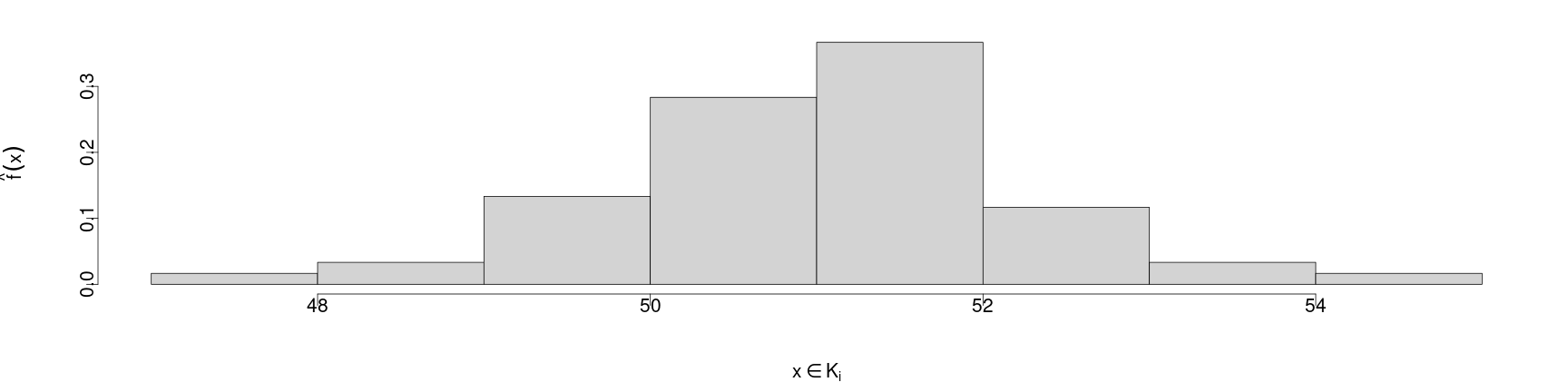### Histograms

• In the following example, breaks = seq(47, 55, 0.5) means that the histogram should range from 47 to 55 with a break every 0.5 step, i.e. $K_1 = [47, 47.5), K 2 = [47.5, 48), ....$
par(cex = 1.75, mai=c(1.5,1.5,.5,.5), mgp=c(3,0,0))
hist(nhtemp, freq = F, breaks = seq(from = 47, to = 55, by = 0.5), main = "", ylab = expression(hat(f)(x)), xlab = expression(x %in% K[i]))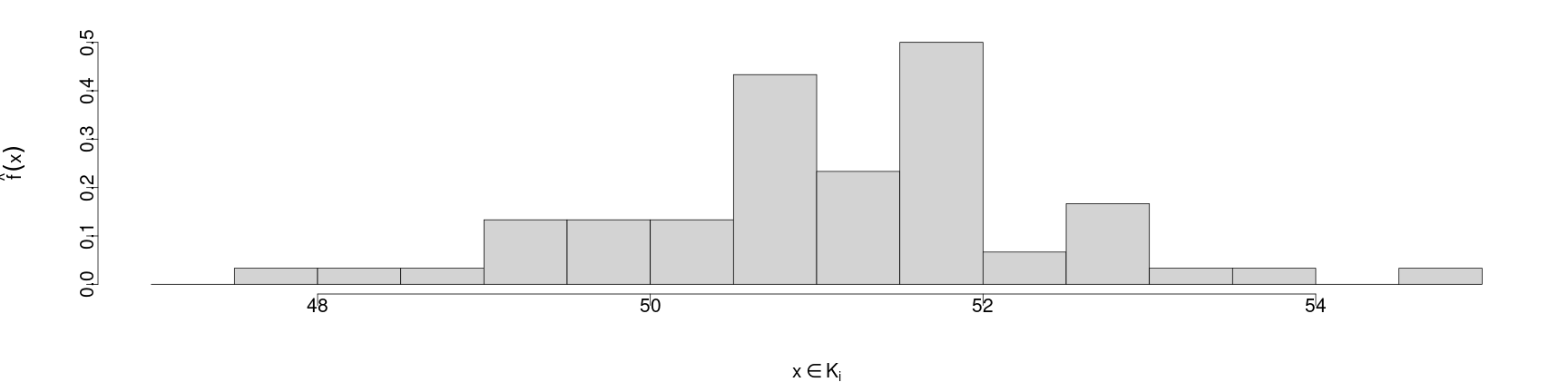### Kernel density estimators

• The histogram is a density estimator with a relatively low rate of convergence to the true density.

• A simple idea to improve the rate of convergence is to use a function that weights the observations in the vicinity of the point where we want to estimate the density, depending on how far away each such observation is from that point.

• Therefore, the kernel estimated density is defined as \begin{align} \hat{f}_h (x) = \frac{1}{n}\sum_{i=1}^n K_h(x - x_i) = \frac{1}{hn}\sum_{i=1}^n K\left(\frac{x-x_i}{h} \right) \end{align}

• The $$K_h(x−x_i)$$ is the kernel, which is a symmetric nonnegative real valued integrable function.

• The expression above can be considered similar to the histogram, but by choosing one of a class of possible weight functions $$K_h$$;
• the weight that $$K_h$$ gives values is according to the distance of the argument $$x$$ from the $$i$$-th observation $$x_i$$ weighted by the “bandwidth” $$h$$.
• Furthermore, the kernel should have the following properties:

1. $$\int_{-\infty}^\infty uK(u)\mathrm{d}u = 0$$;
2. $$\int_{-\infty}^\infty k(u) \mathrm{d}u = 1$$.